# Angle Measurement for Pizza-Crust Lovers

Whether we measure a right angle to be 90 degrees or 100 grads, either way we are adopting an arbitrary scale.  But when we say that its measure is π/2 radians, we are using a system that is not arbitrary at all.  It is based on a geometric comparison that works a lot like an aspect ratio.

As we said earlier, the aspect ratio of a rectangle is the ratio of length to width.  Intuitively, it is a measure of the “rectangularity”.  Long skinny rectangles have high aspect ratios.  Squares have aspect ratio L/W = 1.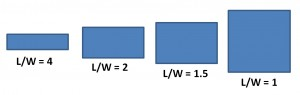One important thing to understand about aspect ratios: they don’t tell you anything about the size of the rectangle.  These two rectangles both have the same aspect ratio (2.5) though one is clearly bigger: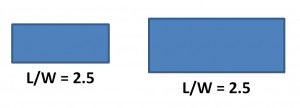Aspect ratios tell you about the shape, not the size.

Also, as long as you measure the length and width with the same units, the aspect ratio is “dimensionless”.  For a given rectangle, you get the same aspect ratio whether you measure your length and width in inches, feet, yards, miles…it doesn’t matter, as long as you use the same units for both measurements.

Onward to Angle Measurement

Here again are a collection of slices all cut from the same pizza but with different central angles: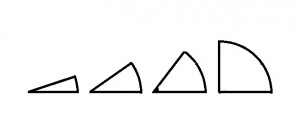And here’s a critical fact to notice about these slices: for pizza of a given radius, the bigger the angle, the more crust you get.  (Many of my mathematical insights involve food. Why is that?)

The crust length is better known as “arc length”.  And now we can define a kind of aspect ratio.  Instead of length-to-width, let’s base this aspect ratio on the arc length and the radius.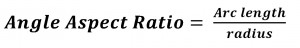The arc length-to-radius ratio is a natural and completely non-arbitrary way to measure the angles.  All of the slices above have the same radius.  So the biggest arc length-to-radius ratio is found on the right where the crust is longest.  And that slice has the biggest central angle.

For example, suppose you cut two slices from a pizza with a radius of 12 inches, one with 6 inches of crust and the other with 9 inches of crust.

We can define the measure of the central angle as the Angle Aspect Ratio.

For the smaller piece…

Angle Aspect Ratio = Arc Length / Radius = 6inches / 12 inches = 1/2.

And for the larger piece…

Angle Aspect Ratio = Arc Length / Radius = 9inches / 12 inches = 3/4.

As expected, the larger Angle Aspect Ratio is associated with the larger central angle.  You got more crust per radius with that angle.

Also, just like aspect ratios for rectangles, it’s the shape and not the size that matters. These two slices have the same angular aspect ratio.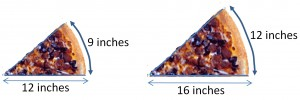The bigger slice has more crust but the crust-to-radius ratio is the same. So both of these angles have the same measure:And yes, the real name for this system is called “radian measure” (and not “angle aspect ratio”, alas).

In this system, the size of a right angle is not an arbitrary 90 degrees or 100 grads.  Instead, it is the ratio of the arc length to the radius.  But we can figure out that value.  We know a full circle has a circumference of 2πr.  So the arc length of a quarter circle must be 2πr ÷ 4 =πr/2.Then, to find the radian measure, we calculate the “angle aspect ratio” (as I stubbornly call it):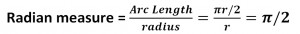And now that we know that a right angle measures π/2 radians, we can also say that